×#### Thank you for registering.

One of our academic counsellors will contact you within 1 working day.

Click to Chat

1800-1023-196

+91-120-4616500

CART 0

• 0

MY CART (5)

Use Coupon: CART20 and get 20% off on all online Study Material

ITEM
DETAILS
MRP
DISCOUNT
FINAL PRICE
Total Price: Rs.

There are no items in this cart.
Continue Shopping• Complete JEE Main/Advanced Course and Test Series
• OFFERED PRICE: Rs. 15,900
• View Details

```Chapter 12: Percentage Exercise – 12.2

Question: 1

Percentage of

1) 22% of 120

2) 25% of 1000

3) 25% of 10 kg

4) 16.5 % of 5000 metre

5) 135 % of 80 cm

6) 2.5 % of 10000 ml

Solution:Question: 2

1) 8.4% of a is 42

2) 0.5% of a is 3

3) (1/2) % of a is 50

4) 100% of a is 100

Solution: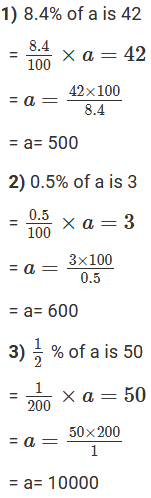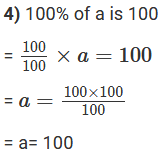Question: 3

x is 5% of y, y is 24% of z. if x = 480, find the values of y and z.

Solution:

Given,

= x is 5% of y

= x= (5/100) y

= y = (100/5) x

= y = 20 x

= y = 20(480)

= y = 9600

Also given,

= y is 24% of z

= y= 24/100 z

= z= 100/24 y

= z = (100/24) × 9600

= z = 40000

Question: 4

A coolie deposits Rs.150 per month in his post office savings bank account. If this is 15% of this monthly income, find his monthly income.

Solution:

Let his monthly income be x

According to the question,

= 15 % of x = 150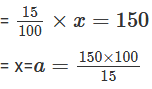= x = 1000

His monthly income is Rs.1000

Question: 5

Asha got 86.875 % marks in the annual examination. If she got 695 marks, find the total number of marks of the examination.

Solution:

Let x be the total number of marks in the examination

According to the question,

= 86.875% of x = 695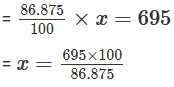= 800

The total number of marks in the examination is 800.

Question: 6

Deepti went to school for 216 days in a full year. If her attendance is 90%, find her number of days on which the school was opened?

Solution:

Let the school was opened for x days

According to the question,

= 90 % of x = 216= x = 240 days

The school was opened for 240 days in the given year.

Question: 7

A garden has 2000 trees. 12% of these are mango trees, 18% are lemon and the rest are orange trees. Find the number of orange trees?

Solution:

Let the number of orange trees be x

There are total 2000 trees.

According to the question,

12% of total trees are mango

Number of mango trees = 12% of 2000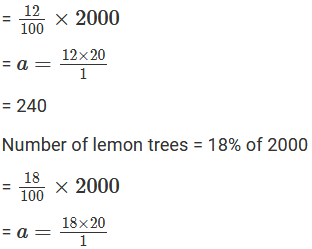= 360

Number of orange trees = (2000 – 240 - 360)

= 1400

Number of orange trees are 1400.

Question: 8

Balanced diet should contain 12% of proteins, 25% of fats and 63% of carbohydrates. If a child needs 2600 calories in this food daily, find in calories the amount of each of these in his daily food intake?

Solution:

In a balanced diet of 2600 calories

12 % protein. Amount of protein intake = 12% of 2600 = (12/100) × 2600 = 312 calories

25 % fats. Amount of fats intake = 25% of 2600 = (25/100) × 2600 = 650 calorie

Amount of carbohydrates intake = 2600 - (315 + 650) = 1638 calories

Question: 9

A cricketer diet scored a total of 62 runs in 96 balls. He hit 3 sixes, 8 fours, 2 twos and 8 singles. What is the percentage of total runs came in:

1) Sixes

2) Fours

3) Twos

4) Singles

Solution: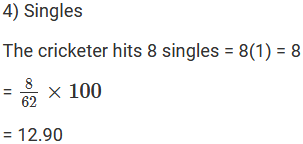Question: 10

A cricketer hits 120 runs in 150 balls during a test match. 20% of the runs came in 6’s. 30% in 4’s. 25% in 2’s. And rest in 1’s. How many rubs did he score in?

Solution:

Let us assume the cricketer scored w runs in 6’s.

20% of 120 = w

= w = 24

Let us assume the cricketer scored x runs in 4’s.

30% of 120 = x

= x = 36

Let us assume the cricketer scored w runs in 2’s.

25% of 120 = y

= y = 30

Let us assume the cricketer scored z runs in 1’s.

24 + 36 + 30 + z = 120

= z = 30

The cricketer scored 30 runs by taking singles.

Question: 11

Radha earns 22% of her investment. If she earns Rs. 187, then how much did she invest?

Solution:

Let the investment be Rs. x

According to the question

22% of x = 187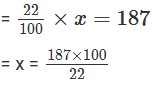= x = 850

Question: 12

Rohit deposits 12% of his income in a bank. He deposited Rs. 1440 in the bank during 1997. What was his total income for the year 1997?

Solution:

Let the total income of the year 1997 be Rs .x

According to the question,

12% of x = 1440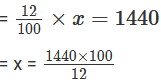= x = 12000

Rohit’s total income during 1997 is Rs.12, 000.

Question: 13

Gun powder contains 75% nitre and 10% sulphur. Find the amount of the gun powder which carries 9 kg of nitre. What amount of gun powder would contain 2.3 kg of sulphur?

Solution:

Let the amount of gun powder that contains 9 kg nitre be x kgLet the amount of gun powder that contains 2.3 kg sulphur be y kg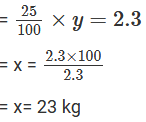The amount of gun powder containing 2.3 kg sulphur is 23 kg.

Question: 14

An alloy of tin and copper consists of 15 parts of tin and 105 parts of copper. Find the percentage of copper in the alloy?

Solution:

Composition of the alloy = 15 parts if tin + 105 parts of copper

Therefore, percentage of tin = Let the amount of gun powder that contains 9 kg nitre be x kg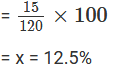Percentage of copper =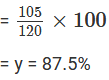The percentage of copper is 87.5%

Question: 15

An alloy contains 32% of copper, 40% of nickel and rest zinc. Find the mass of the zinc in 1 kg of alloy?

Solution:

Percentage of copper in the alloy = 32%

Percentage of nickel in the alloy = 40%

Percentage of zinc in the alloy = 100 - (32 + 40) = 28%

Amount of zinc in 1 kg of alloy = 0.28(1) = 280 gm

The mass of zinc in 1 kg of the alloy is 280 gm.

Question: 16

A motorist travelled 122 kilometres before his first stop. If he had 10% of his journey to complete at this point, how long was the total ride?

Solution:

Let the length of the total ride be x km

According to the question

10% of x = 122= x = 1220 km

The total length of the total ride is 1220 km.

Question: 17

A certain school has 30 students, 142 of whom are boys. It has 30 teachers, 12 of which are men. What percent of total number of students and teachers in the school is female?

Solution:

Total number of female students = 300 - 142 = 158

Number of female teachers = 30 - 12 = 18

To tal number of females = 158 + 18 = 176

Total population of the school = 300 + 30 = 330

Percentage of teacher in the school is female is = (176/330) × 100

= 53.33%

The percentage of total number of students and teachers in the school is female is 53.33%

Question: 18

Aman’s income is 20% less than that of anil. How much present is anil’s income more than aman’s?

Solution:

Let anil’s income be x

Then, aman’s income = (x − 20)100 = 8 × 10

Difference in the incomes of anil and aman to that of aman’s income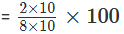= 25%

Anil’s income is 25% more than that of aman’s.

Question: 19

The value of the machine depreciates every year by 5%. If the present value of the machine is Rs.100000, what will be it’s value after 2 years?

Solution:

It is given that the value of the machine depreciates by 5% every year. Present value of the machine = Rs.100000

Therefore, 5% of 100000 = Rs.5000

Value of the machine after 1st year = Rs (100000 - 5000)

= Rs. 95000

5 % of 95000 = Rs.4750

Value of the machine in the 2nd year =Rs (95000 - 4750)

= Rs 90250

After two years, the value of the machine will be Rs.90250

Question: 20

The population of the town increased by 10% annually. If the present population is 60000, what will be its population after 2 years?

Solution:

Present population = 60000

It increases 10% annually

Increase in the population in the first year = 60000 + 6000 = 66000

66000 is the increase in the population in the second year = 10% of 66000 = 6600

Thus population after 2 years = 66000 + 6600 = 72600

The population of the town after 2 years is 72600.

Question: 21

The population of the town is increased by 10% annually. If the present population is 22000, find the population a year ago.

Solution:

Let the population of the town one year ago be x

Now, it is given that population of the town increases by 10%

Present population = x + 10% of x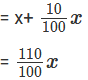But present population of the town = 22000

According to the question,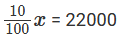= x = 20000

The population of the town a year ago is 20000.

Question: 22

Ankit was given an increment of 10% on his salary. His new salary is Rs. 3575. what was his salary before investment?

Solution:

Let the initial salary be Rs. x

We know that salary

Before increment + increment given on salary = new salary

= x +10% of x = 3575= x = 3250

Salary before increment is Rs.3250

Question: 23

In new budget, the price of the petrol rose by 10%. By how much percent must one reduce the consumption so that the expenditure does not increase?

Solution:

We have to reduce the consumption such that the expenditure does not increase.

For this, we use the following formula: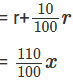Where r is the percentage rise in the price of the commodity.

Therefore, percentage reduction in the consumption = 9111

Question: 24

Mohan’s income is Rs.15500 per month. He saves 11% of his income. If his income is increased by 10%, then he reduces his saving by 1%, how much does he save now?

Solution: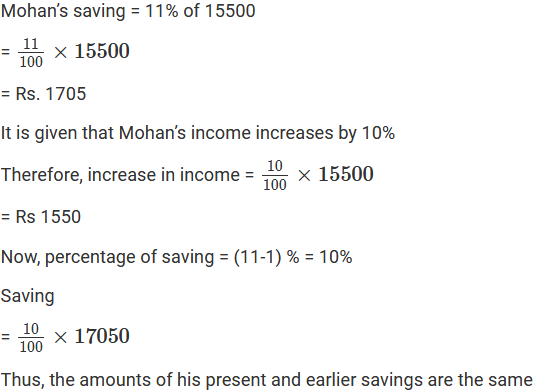Question: 25

Shikha’s income is 60% more than that of shalu. What percent is shalu’s income less than shikha’s?

Solution:

Let shalu’s income be Rs.x

Shikha’s income = Rs x + 60% of xPercentage of the difference in the incomes of shikha and shalu to that of shikha’s income = 37.5%

Question: 26

Rs.3500 is to be shared among three people so that the first person gets 50% of the second, who in turn gets 50% of the third. How much will each other of them get?

Solution:

Let x, y, z be the the amounts received by the first, second, third person respectively.Question: 27

After a 20% hike, the cost of Chinese vase is Rs2000. What was the original price of the object?

Solution:```### Course Features

• 728 Video Lectures
• Revision Notes
• Previous Year Papers
• Mind Map
• Study Planner
• NCERT Solutions
• Discussion Forum
• Test paper with Video Solution